# 用电量

``````
plot (ts( data = Load , start= 1996 , frequency = 52) )``````

•星期几（离散）
•时间小时（离散或非参数）
•年（连续）

•日期和时间

•年份和时间

•公共假期

GAM模型中的样条曲线

R语言广义相加模型（GAM）在电力负荷预测中的应用

# 数据探索

``> plot ( NumWeek , Load )``

``> plot ( Temp , Load )``

``````> acf (Load )
``````

# OLS与 中位数回归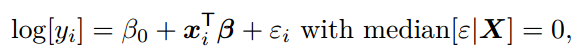``````lm(y˜x, data =df)
lm(y˜x, data =df , tau =.5)``````

# 现在，中位数回归将始终有两个观察结果。

``````
which ( predict ( fit ))
21 46``````

# 分位数回归和指数平滑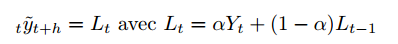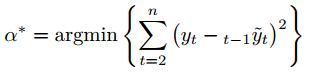``````
X=as. numeric ( Nile )
SimpleSmooth = function (a){
for (t in 2:T{L[t=a*X[t+(1 -a)*L[t -1
}lines ( SimpleSmooth (.2) ,col =" red ")``````
``````V= function (a){

for (t in 2:T){
L[t]=a*X[t]+(1 -a)*L[t -1]
erreur [t]=X[t]-L[t -1] }
return ( sum ( erreur ˆ2) )

optim (.5 ,V)\$ par
 0.2464844
hw= HoltWinters (X, beta =FALSE
hw\$ alpha
 0.2465579``````

### 随时关注您喜欢的主题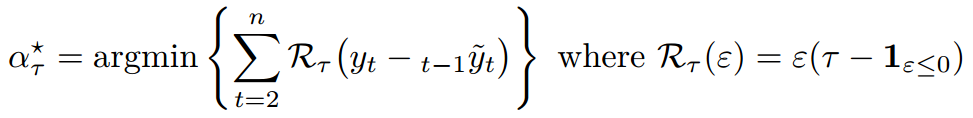``````HWtau = function ( tau ){
loss = function (e) e*(tau -(e< ;=0) *1)
V= function (a){

for (t in 2:T){
L[t]=a*X[t+(1 -a)*L[t -1
erreur [t=X[t-L[t -1
return ( sum ( loss ( erreur

optim (.5 ,V)\$ par
``````

``````plot (X, type ="b",cex =.6

lines ( SimpleSmooth ( HWtau (.8,col=" blue ",
lwd =2)``````

# 双指数平滑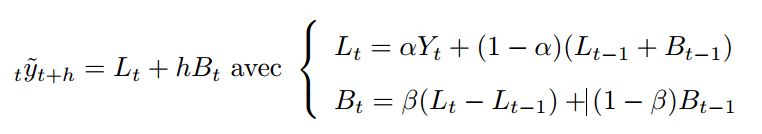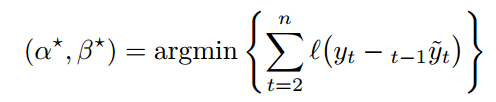``````
hw= HoltWinters (X, gamma =FALSE ,l. start =X)
hw\$ alpha
alpha
0.4223241
hw\$ beta
beta
0.05233389

DouSmo = function (a,b){

for (t in 2:T){
L[t]=a*X[t+(1 -a*(L[t -1]+ B[t -1]
B[t]=b*(L[t]-L[t -1]) +(1 -b*B[t -1]
return (L+B)``````

# 预测变量的构造

``````
1996 , frequency = 52) ,col =" white "``````

# 回归

``````plot (ts( data = Temp , start =
1996 , frequency = 52) ,
lines (ts( data = train \$Temp , start =
1996 , frequency = 52) )
lines (ts( data = test \$Temp , start =
1996+620 /52, frequency = 52) ``````

# SARIMA模型

s = 52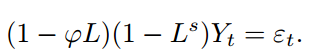``````
ARIMA = arima (z, order =c(1 ,0 ,0 ,seasonal =list ( order =c(0 ,1 ,0 ,period =52
plot ( forecast (ARIMA ,h =112 )``````

Kaizong Ye拓端研究室（TRL）的研究员。

​非常感谢您阅读本文，如需帮助请联系我们！QQ在线咨询

15121130882

0571-63341498

## 关注有关新文章的微信公众号

This will close in 0 seconds